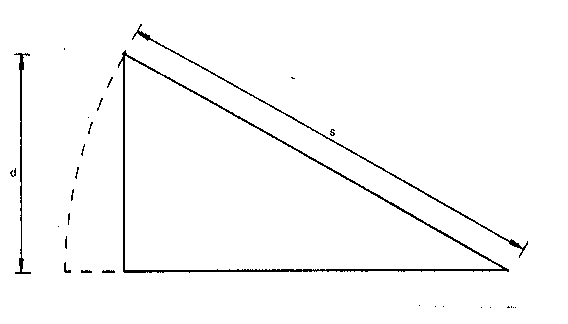Slope Correction
When you tape a distance on a slope, even a gentle one, the measured distance given by the tape is the ground distance, and because of the slope, this is slightly longer than the required plan distance. To correct this, you need to know the difference in height between the two points on a slope.From the diagram, it can be seen that Ground distance L, plan distance h, and height difference d are 3 sides of a right-angled triangle. Thereforeh (squared) = L (squared) - d (squared)or, h = L (multiplied by) cos theta

Subtract L from h to give the correction to be applied.